2021-9-27 16:52
27679

# AFL二三事 -- 源码分析 2

## 一、LLVM 前置知识

LLVM 主要为了解决编译时多种多样的前端和后端导致编译环境复杂、苛刻的问题，其核心为设计了一个称为 `LLVM IR` 的中间表示，并以库的形式提供一些列接口，以提供诸如操作 IR 、生成目标平台代码等等后端的功能。其整体架构如下所示：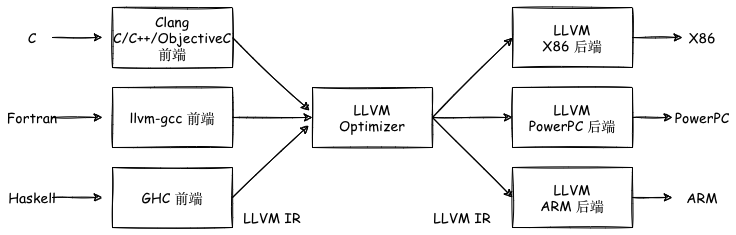GCC 的前后端耦合在一起，没有进行分离，所以GCC为了支持一门新的编程语言或一个新的硬件设备，需要重新开发前端到后端的完整过程。

Clang 是 LLVM 项目的一个子项目，它是 LLVM 架构下的 C/C++/Objective-C 的编译器，是 LLVM 前端的一部分。相较于GCC，具备编译速度快、占用内存少、模块化设计、诊断信息可读性强、设计清晰简单等优点。（LLVM Pass 是一些中间过程处理 IR 的可以用户自定义的内容，可以用来遍历、修改 IR 以达到插桩、优化、静态分析等目的。）

## 二、 AFL的afl-clang-fast

### 1. 概述

AFL的 `llvm_mode` 可以实现编译器级别的插桩，可以替代 `afl-gcc``afl-clang` 使用的比较“粗暴”的汇编级别的重写的方法，且具备如下几个优势：

1. 编译器可以进行很多优化以提升效率；
2. 可以实现CPU无关，可以在非 x86 架构上进行fuzz；
3. 可以更好地处理多线程目标。

`afl-llvm-rt.o.c` 文件主要是重写了 `afl-as.h` 文件中的 `main_payload` 部分，方便调用；

`afl-llvm-pass.so.cc` 文件主要是当通过 `afl-clang-fast` 调用 clang 时，这个pass被插入到 LLVM 中，告诉编译器添加与 ``afl-as.h` 中大致等效的代码；

`afl-clang-fast.c` 文件本质上是 clang 的 wrapper，最终调用的还是 clang 。但是与 `afl-gcc` 一样，会进行一些参数处理。

`llvm_mode` 的插桩思路就是通过编写pass来实现信息记录，对每个基本块都插入探针，具体代码在 `afl-llvm-pass.so.cc` 文件中，初始化和forkserver操作通过链接完成。

### 2. 源码

#### 1. afl-clang-fast.c

##### 1. main 函数

`main` 函数的全部逻辑如下：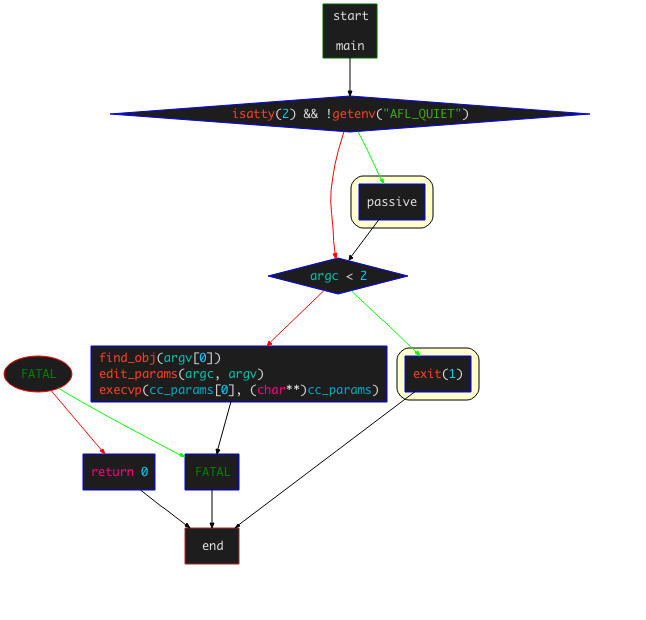• `find_obj(argv)`：查找运行时library
• `edit_params(argc, argv)`：处理传入的编译参数，将确定好的参数放入 `cc_params[]` 数组
• `execvp(cc_params, (cahr**)cc_params)`：替换进程空间，传递参数，执行要调用的clang

##### 2. find_obj 函数

`find_obj()`函数的控制流逻辑如下：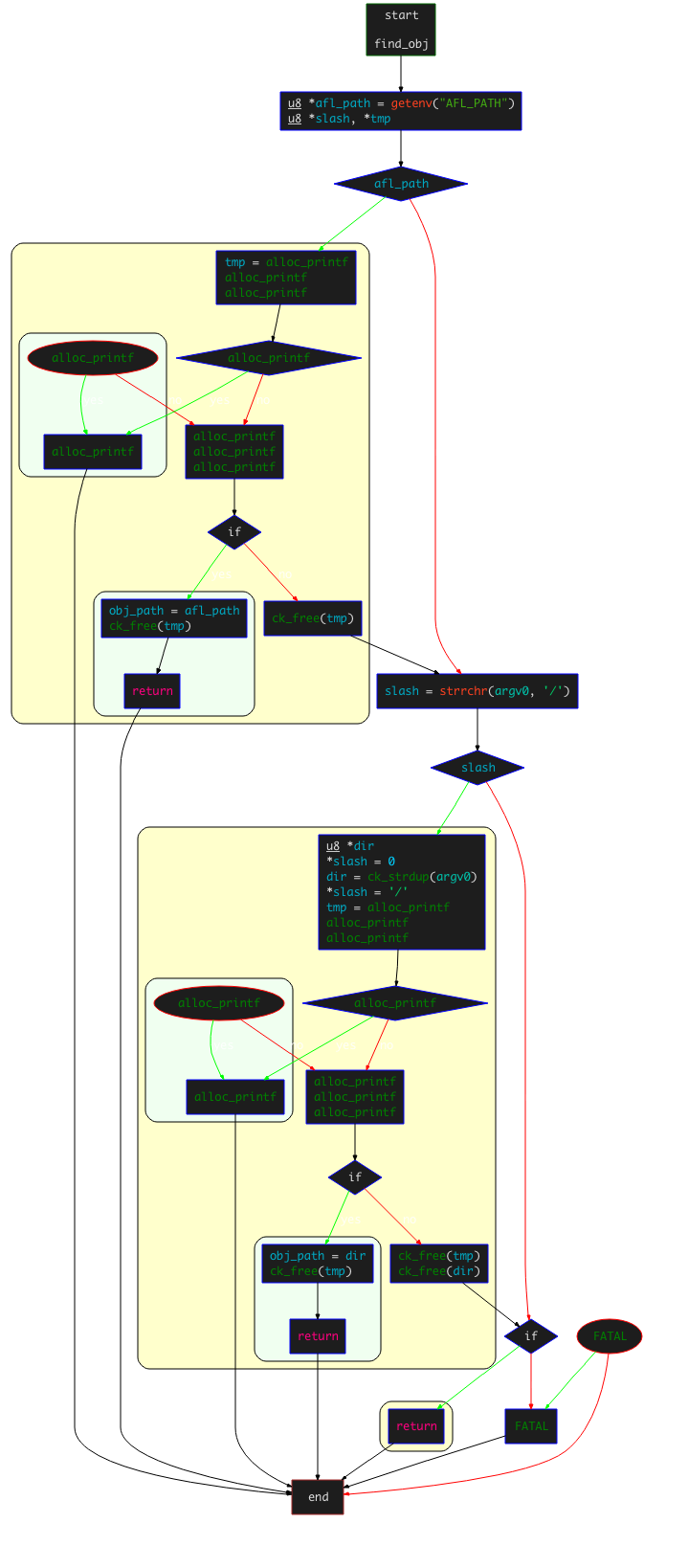• 首先，读取环境变量 `AFL_PATH` 的值：
• 如果读取成功，确认 `AFL_PATH/afl-llvm-rt.o` 是否可以访问；如果可以访问，设置该目录为 `obj_path` ，然后直接返回；
• 如果读取失败，检查 `arg0` 中是否存在 `/` 字符，如果存在，则判断最后一个 `/` 前面的路径为 AFL 的根目录；然后读取`afl-llvm-rt.o`文件，成功读取，设置该目录为 `obj_path` ，然后直接返回。
• 如果上面两种方式都失败，到`/usr/local/lib/afl` 目录下查找是否存在 `afl-llvm-rt.o` ，如果存在，则设置为 `obj_path` 并直接返回（之所以向该路径下寻找，是因为默认的AFL的MakeFile在编译时，会定义一个名为`AFL_PATH`的宏，该宏会指向该路径）；
• 如果以上全部失败，抛出异常提示找不到 `afl-llvm-rt.o` 文件或 `afl-llvm-pass.so` 文件，并要求设置 `AFL_PATH` 环境变量 。

##### 3. edit_params 函数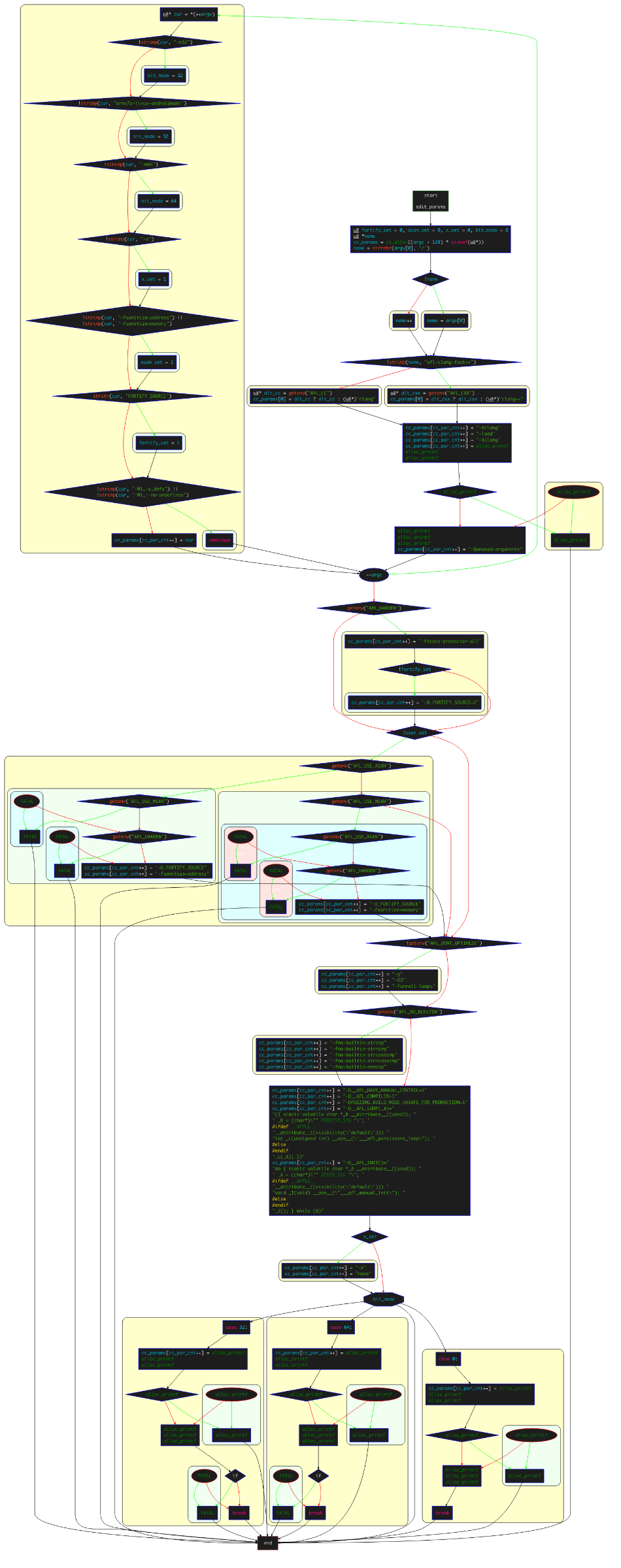• 首先，判断执行的是否为 `afl-clang-fast++`

• 如果是，设置 `cc_params` 为环境变量 `AFL_CXX`；如果环境变量为空，则设置为 `clang++`
• 如果不是，设置 `cc_params` 为环境变量 `AFL_CC`；如果环境变量为空，则设置为 `clang`
• 判断是否定义了 `USE_TRACE_PC` 宏，如果有，添加 `-fsanitize-coverage=trace-pc-guard -mllvm(only Android) -sanitizer-coverage-block-threshold=0(only Android)` 选项到参数数组；如果没有，依次将 `-Xclang -load -Xclang obj_path/afl-llvm-pass.so -Qunused-arguments` 选项添加到参数数组；（这里涉及到llvm_mode使用的2种插桩方式：默认使用的是传统模式，使用 `afl-llvm-pass.so` 注入来进行插桩，这种方式较为稳定；另外一种是处于实验阶段的方式——`trace-pc-guard` 模式，对于该模式的详细介绍可以参考llvm相关文档——tracing-pcs-with-guards

• 遍历传递给 `afl-clang-fast` 的参数，进行一定的检查和设置，并添加到 `cc_params` 数组：

• 如果存在 `-m32``armv7a-linux-androideabi` ，设置 `bit_mode` 为32；
• 如果存在 `-m64` ，设置 `bit_mode` 为64；
• 如果存在 `-x` ，设置 `x_set` 为1；
• 如果存在 `-fsanitize=address``-fsanitize=memory`，设置 `asan_set` 为1；
• 如果存在 `-Wl,-z,defs``-Wl,--no-undefined`，则直接pass掉。
• 检查环境变量是否设置了 `AFL_HARDEN`

• 如果有，添加 `-fstack-protector-all` 选项；
• 如果有且没有设置 `FORTIFY_SOURCE` ，添加 `-D_FORTIFY_SOURCE=2` 选项；
• 检查参数中是否存在 `-fsanitize=memory`，即 `asan_set` 为0：

• 如果没有，尝试读取环境变量 `AFL_USE_ASAN`，如果存在，添加 `-U_FORTIFY_SOURCE -fsanitize=address`
• 接下来对环境变量`AFL_USE_MSAN`的处理方式与 `AFL_USE_ASAN` 类似，添加的选项为 `-U_FORTIFY_SOURCE -fsanitize=memory`
• 检查是否定义了 `USE_TRACE_PC` 宏，如果存在定义，检查是否存在环境变量 `AFL_INST_RATIO`，如果存在，抛出异常`AFL_INST_RATIO` 无法在trace-pc时使用；

• 检查环境变量 `AFL_NO_BUILTIN` ，如果没有设置，添加 `-g -O3 -funroll-loops`
• 检查环境变量 `AFL_NO_BUILTIN`，如果进行了设置，添加 `-fno-builtin-strcmp -fno-builtin-strncmp -fno-builtin-strcasecmp -fno-builtin-strcasecmp -fno-builtin-memcmp`
• 添加参数 `-D__AFL_HAVE_MANUAL_CONTROL=1 -D__AFL_COMPILER=1 -DFUZZING_BUILD_MODE_UNSAFE_FOR_PRODUCTION=1`
• 定义了两个宏 `__AFL_LOOP(), __AFL_INIT()`
• 检查是否设置了 `x_set`， 如果有添加 `-x none`
• 检查是否设置了宏 `__ANDORID__` ，如果没有，判断 `bit_mode` 的值：
• 如果为0，即没有`-m32``-m64`，添加 `obj_path/afl-llvm-rt.o`
• 如果为32，添加 `obj_path/afl-llvm-rt-32.o`
• 如果为64，添加 `obj_path/afl-llvm-rt-64.o`

#### 2. afl-llvm-pass.so.cc

`afl-llvm-pass.so.cc` 文件实现了 LLVM-mode 下的一个插桩 LLVM Pass。

 123456789101112131415161718 `namespace {` `  ``class` `AFLCoverage : public ModulePass {` `    ``public:` `      ``static char ``ID``;``      ``AFLCoverage() : ModulePass(``ID``) { }` `      ``bool` `runOnModule(Module &M) override;` `      ``/``/` `StringRef getPassName() const override {``      ``/``/`  `return` `"American Fuzzy Lop Instrumentation"``;``      ``/``/` `}` `  ``};` `}`
##### 1. pass注册

 12345678910111213 `static void registerAFLPass(const PassManagerBuilder &,``                            ``legacy::PassManagerBase &PM) {` `  ``PM.add(new AFLCoverage());` `}`  `static RegisterStandardPasses RegisterAFLPass(``    ``PassManagerBuilder::EP_ModuleOptimizerEarly, registerAFLPass);` `static RegisterStandardPasses RegisterAFLPass0(``    ``PassManagerBuilder::EP_EnabledOnOptLevel0, registerAFLPass);`

##### 2. runOnModule 函数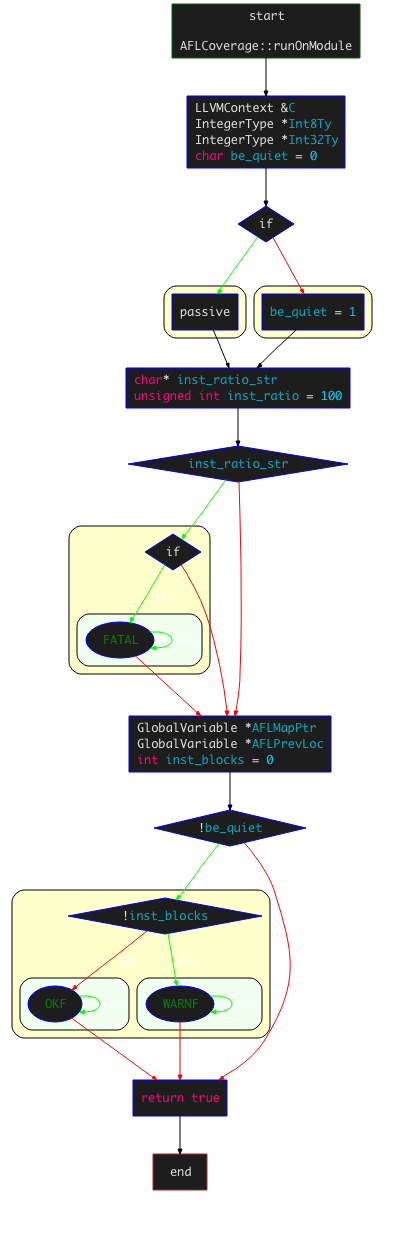• 首先，通过 `getContext()` 来获取 `LLVMContext` ，获取进程上下文：

 1234 `LLVMContext &C ``=` `M.getContext();` `IntegerType ``*``Int8Ty  ``=` `IntegerType::getInt8Ty(C);``IntegerType ``*``Int32Ty ``=` `IntegerType::getInt32Ty(C);`
• 设置插桩密度：读取环境变量 `AFL_INST_RATIO` ，并赋值给 `inst_ratio`，其值默认为100，范围为 1～100，该值表示插桩概率；

• 获取只想共享内存shm的指针以及上一个基本块的随机ID：

 1234567 `GlobalVariable ``*``AFLMapPtr ``=``  ``new GlobalVariable(M, PointerType::get(Int8Ty, ``0``), false,``                     ``GlobalValue::ExternalLinkage, ``0``, ``"__afl_area_ptr"``);` `GlobalVariable ``*``AFLPrevLoc ``=` `new GlobalVariable(``  ``M, Int32Ty, false, GlobalValue::ExternalLinkage, ``0``, ``"__afl_prev_loc"``,``  ``0``, GlobalVariable::GeneralDynamicTLSModel, ``0``, false);`
• 进入插桩过程：

• 通过 `for` 循环遍历每个BB（基本块），寻找BB中适合插入桩代码的位置，然后通过初始化 `IRBuilder` 实例执行插入；

 12 `BasicBlock::iterator IP ``=` `BB.getFirstInsertionPt();``      ``IRBuilder<> IRB(&(``*``IP));`

 12345678910111213 `if` `(AFL_R(``100``) >``=` `inst_ratio) ``continue``; ``/``/` `如果大于插桩密度，进行随机插桩` `/``*` `Make up cur_loc ``*``/` `unsigned ``int` `cur_loc ``=` `AFL_R(MAP_SIZE);` `ConstantInt ``*``CurLoc ``=` `ConstantInt::get(Int32Ty, cur_loc);  ``/``/` `随机创建当前基本块``ID` `/``*` `Load prev_loc ``*``/` `LoadInst ``*``PrevLoc ``=` `IRB.CreateLoad(AFLPrevLoc);``PrevLoc``-``>setMetadata(M.getMDKindID(``"nosanitize"``), MDNode::get(C, ``None``));``Value ``*``PrevLocCasted ``=` `IRB.CreateZExt(PrevLoc, IRB.getInt32Ty()); ``/``/` `获取上一个基本块的随机``ID`
• 插入load指令，获取共享内存的地址，并调用 `CreateGEP` 函数获取共享内存中指定index的地址；

 123456 `/``*` `Load SHM pointer ``*``/` `LoadInst ``*``MapPtr ``=` `IRB.CreateLoad(AFLMapPtr);``MapPtr``-``>setMetadata(M.getMDKindID(``"nosanitize"``), MDNode::get(C, ``None``));``Value ``*``MapPtrIdx ``=``  ``IRB.CreateGEP(MapPtr, IRB.CreateXor(PrevLocCasted, CurLoc));`

 1234567 `/``*` `Update bitmap ``*``/` `LoadInst ``*``Counter ``=` `IRB.CreateLoad(MapPtrIdx);``Counter``-``>setMetadata(M.getMDKindID(``"nosanitize"``), MDNode::get(C, ``None``));``Value ``*``Incr ``=` `IRB.CreateAdd(Counter, ConstantInt::get(Int8Ty, ``1``));``IRB.CreateStore(Incr, MapPtrIdx)``        ``-``>setMetadata(M.getMDKindID(``"nosanitize"``), MDNode::get(C, ``None``));`
• 右移 `cur_loc` ，插入store指令，更新 `__afl_prev_loc`

 12345 `/``*` `Set` `prev_loc to cur_loc >> ``1` `*``/` `StoreInst ``*``Store ``=``  ``IRB.CreateStore(ConstantInt::get(Int32Ty, cur_loc >> ``1``), AFLPrevLoc);``Store``-``>setMetadata(M.getMDKindID(``"nosanitize"``), MDNode::get(C, ``None``));`
• 最后对插桩计数加1；

• 扫描下一个BB，根据设置是否为quiet模式等，并判断 `inst_blocks` 是否为0，如果为0则说明没有进行插桩；

 12345678 `if` `(!be_quiet) {` `  ``if` `(!inst_blocks) WARNF(``"No instrumentation targets found."``);``  ``else` `OKF(``"Instrumented %u locations (%s mode, ratio %u%%)."``,``           ``inst_blocks, getenv(``"AFL_HARDEN"``) ? ``"hardened"` `:``           ``((getenv(``"AFL_USE_ASAN"``) || getenv(``"AFL_USE_MSAN"``)) ?``            ``"ASAN/MSAN"` `: ``"non-hardened"``), inst_ratio);``}`

##### 3. afl-llvm-rt.o.c

###### 1. deferred instrumentation

AFL会尝试通过只执行一次目标二进制文件来提升性能，在 `main()` 之前暂停程序，然后克隆“主”进程获得一个稳定的可进行持续fuzz的目标。简言之，避免目标二进制文件的多次、重复的完整运行，而是采取了一种类似快照的机制。

 123 `#ifdef __AFL_HAVE_MANUAL_CONTROL``    ``__AFL_INIT();``#endif`

 12345678910 `  ``cc_params[cc_par_cnt``+``+``] ``=` `"-D__AFL_INIT()="``    ``"do { static volatile char *_A __attribute__((used)); "``    ``" _A = (char*)\""` `DEFER_SIG ``"\"; "``#ifdef __APPLE__``    ``"__attribute__((visibility(\"default\"))) "``    ``"void _I(void) __asm__(\"___afl_manual_init\"); "``#else``    ``"__attribute__((visibility(\"default\"))) "``    ``"void _I(void) __asm__(\"__afl_manual_init\"); "``#endif /* ^__APPLE__ */`

`__afl_manual_init()` 函数实现如下：

 12345678910111213141516 `/``*` `This one can be called ``from` `user code when deferred forkserver mode``    ``is` `enabled. ``*``/` `void __afl_manual_init(void) {` `  ``static u8 init_done;` `  ``if` `(!init_done) {` `    ``__afl_map_shm();``    ``__afl_start_forkserver();``    ``init_done ``=` `1``;` `  ``}` `}`

 123456789101112131415161718192021222324 `/``*` `SHM setup. ``*``/` `static void __afl_map_shm(void) {` `  ``u8 ``*``id_str ``=` `getenv(SHM_ENV_VAR); ``/``/` `读取环境变量 SHM_ENV_VAR 获取``id` `  ``if` `(id_str) { ``/``/` `成功读取``id` `    ``u32 shm_id ``=` `atoi(id_str);` `    ``__afl_area_ptr ``=` `shmat(shm_id, NULL, ``0``); ``/``/` `获取shm地址，赋给 __afl_area_ptr` `    ``/``*` `Whooooops. ``*``/` `    ``if` `(__afl_area_ptr ``=``=` `(void ``*``)``-``1``) _exit(``1``);  ``/``/` `异常则退出` `    ``/``*` `Write something into the bitmap so that even with low AFL_INST_RATIO,``       ``our parent doesn't give up on us. ``*``/` `    ``__afl_area_ptr[``0``] ``=` `1``; ``/``/` `进行设置` `  ``}` `}`

 123456789101112131415161718192021222324252627282930313233343536373839404142434445464748495051525354555657585960616263646566676869707172737475767778798081 `/``*` `Fork server logic. ``*``/` `static void __afl_start_forkserver(void) {` `  ``static u8 tmp[``4``];``  ``s32 child_pid;` `  ``u8  child_stopped ``=` `0``;` `  ``/``*` `Phone home ``and` `tell the parent that we``'re OK. If parent isn'``t there,``     ``assume we're ``not` `running ``in` `forkserver mode ``and` `just execute program. ``*``/` `  ``if` `(write(FORKSRV_FD ``+` `1``, tmp, ``4``) !``=` `4``) ``return``; ``/``/` `写入``4``字节到状态管道，通知 fuzzer已准备完成` `  ``while` `(``1``) {` `    ``u32 was_killed;``    ``int` `status;` `    ``/``*` `Wait ``for` `parent by reading ``from` `the pipe. Abort ``if` `read fails. ``*``/` `    ``if` `(read(FORKSRV_FD, &was_killed, ``4``) !``=` `4``) _exit(``1``); ` `    ``/``*` `If we stopped the child ``in` `persistent mode, but there was a race``       ``condition ``and` `afl``-``fuzz already issued SIGKILL, write off the old``       ``process. ``*``/` `      ``/``/` `处于persistent mode且子进程已被killed``    ``if` `(child_stopped && was_killed) {``      ``child_stopped ``=` `0``;``      ``if` `(waitpid(child_pid, &status, ``0``) < ``0``) _exit(``1``);``    ``}` `    ``if` `(!child_stopped) { ` `      ``/``*` `Once woken up, create a clone of our process. ``*``/` `      ``child_pid ``=` `fork(); ``/``/` `重新fork``      ``if` `(child_pid < ``0``) _exit(``1``);` `      ``/``*` `In child process: close fds, resume execution. ``*``/` `      ``if` `(!child_pid) {` `        ``close(FORKSRV_FD); ``/``/` `关闭fd，``        ``close(FORKSRV_FD ``+` `1``);``        ``return``;` `      ``}` `    ``} ``else` `{` `      ``/``*` `Special handling ``for` `persistent mode: ``if` `the child ``is` `alive but``         ``currently stopped, simply restart it with SIGCONT. ``*``/` `      ``/``/` `子进程只是暂停，则进行重启``      ``kill(child_pid, SIGCONT);``      ``child_stopped ``=` `0``;` `    ``}` `    ``/``*` `In parent process: write PID to pipe, then wait ``for` `child. ``*``/` `    ``if` `(write(FORKSRV_FD ``+` `1``, &child_pid, ``4``) !``=` `4``) _exit(``1``);` `    ``if` `(waitpid(child_pid, &status, is_persistent ? WUNTRACED : ``0``) < ``0``)``      ``_exit(``1``);` `    ``/``*` `In persistent mode, the child stops itself with SIGSTOP to indicate``       ``a successful run. In this case, we want to wake it up without forking``       ``again. ``*``/` `    ``if` `(WIFSTOPPED(status)) child_stopped ``=` `1``;` `    ``/``*` `Relay wait status to pipe, then loop back. ``*``/` `    ``if` `(write(FORKSRV_FD ``+` `1``, &status, ``4``) !``=` `4``) _exit(``1``);` `  ``}` `}`

• 首先，设置 `child_stopped = 0`，写入4字节到状态管道，通知fuzzer已准备完成；

• 进入 `while` ，开启fuzz循环：

• 调用 `read` 从控制管道读取4字节，判断子进程是否超时。如果管道内读取失败，发生阻塞，读取成功则表示AFL指示forkserver执行fuzz；
• 如果 `child_stopped` 为0，则fork出一个子进程执行fuzz，关闭和控制管道和状态管道相关的fd，跳出fuzz循环；

• 如果 `child_stopped` 为1，在 `persistent mode` 下进行的特殊处理，此时子进程还活着，只是被暂停了，可以通过`kill(child_pid, SIGCONT)`来简单的重启，然后设置`child_stopped`为0；

• forkserver向状态管道 `FORKSRV_FD + 1` 写入子进程的pid，然后等待子进程结束；
• `WIFSTOPPED(status)` 宏确定返回值是否对应于一个暂停子进程，因为在 `persistent mode` 里子进程会通过 `SIGSTOP` 信号来暂停自己，并以此指示运行成功，我们需要通过 `SIGCONT`信号来唤醒子进程继续执行，不需要再进行一次fuzz，设置`child_stopped`为1；
• 子进程结束后，向状态管道 `FORKSRV_FD + 1` 写入4个字节，通知AFL本次执行结束。
###### 2. persistent mode

`persistent mode` 并没有通过fork子进程的方式来执行fuzz。一些库中提供的API是无状态的，或者可以在处理不同输入文件之间进行重置，恢复到之前的状态。执行此类重置时，可以使用一个长期存活的进程来测试多个用例，以这种方式来减少重复的 `fork()` 调用和操作系统的开销。不得不说，这种思路真的很优秀。

 123456789 `while` `(__AFL_LOOP(``1000``)) {` `  ``/``*` `Read ``input` `data. ``*``/``  ``/``*` `Call library code to be fuzzed. ``*``/``  ``/``*` `Reset state. ``*``/` `}` `/``*` `Exit normally ``*``/`

 123456789101112131415161718192021222324252627282930313233343536373839404142434445464748495051 `#include ``#include ``#include ``#include ``#include `  `/``*` `Main entry point. ``*``/` `int` `main(``int` `argc, char``*``*` `argv) {` `  ``char buf[``100``]; ``/``*` `Example``-``only ``buffer``, you'd replace it with other ``global` `or``                    ``local variables appropriate ``for` `your use case. ``*``/` `  ``while` `(__AFL_LOOP(``1000``)) {` `    ``/``*``*``*` `PLACEHOLDER CODE ``*``*``*``/` `    ``/``*` `STEP ``1``: 初始化所有变量 ``*``/` `    ``memset(buf, ``0``, ``100``);` `    ``/``*` `STEP ``2``: 读取输入数据，从文件读入时需要先关闭旧的fd然后重新打开文件``*``/` `    ``read(``0``, buf, ``100``);` `    ``/``*` `STEP ``3``: 调用待fuzz的code``*``/` `    ``if` `(buf[``0``] ``=``=` `'f'``) {``      ``printf(``"one\n"``);``      ``if` `(buf[``1``] ``=``=` `'o'``) {``        ``printf(``"two\n"``);``        ``if` `(buf[``2``] ``=``=` `'o'``) {``          ``printf(``"three\n"``);``          ``if` `(buf[``3``] ``=``=` `'!'``) {``            ``printf(``"four\n"``);``            ``abort();``          ``}``        ``}``      ``}``    ``}` `    ``/``*``*``*` `END PLACEHOLDER CODE ``*``*``*``/` `  ``}` `  ``/``*` `循环结束，正常结束。AFL会重启进程，并清理内存、剩余fd等 ``*``/` `  ``return` `0``;` `}`

 1234567891011 `  ``cc_params[cc_par_cnt``+``+``] ``=` `"-D__AFL_LOOP(_A)="``    ``"({ static volatile char *_B __attribute__((used)); "``    ``" _B = (char*)\""` `PERSIST_SIG ``"\"; "``#ifdef __APPLE__``    ``"__attribute__((visibility(\"default\"))) "``    ``"int _L(unsigned int) __asm__(\"___afl_persistent_loop\"); "``#else``    ``"__attribute__((visibility(\"default\"))) "``    ``"int _L(unsigned int) __asm__(\"__afl_persistent_loop\"); "``#endif /* ^__APPLE__ */``    ``"_L(_A); })"``;`

`__afl_persistent_loop(unsigned int max_cnt)` 的逻辑如下：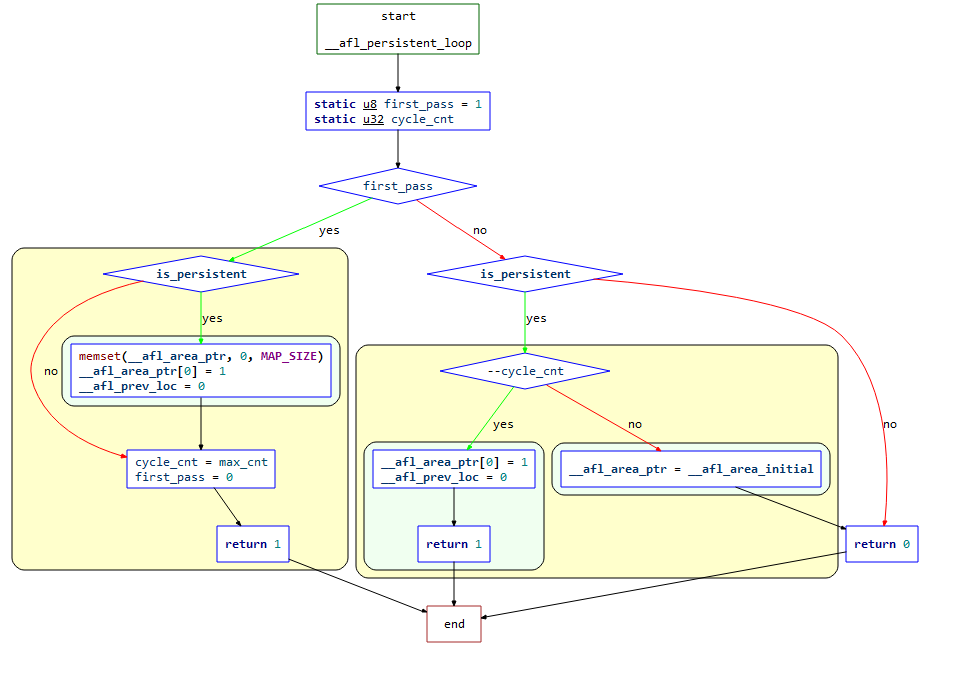1234567891011121314151617181920212223242526272829303132333435363738394041424344 `/``*` `A simplified persistent mode handler, used as explained ``in` `README.llvm. ``*``/` `int` `__afl_persistent_loop(unsigned ``int` `max_cnt) {` `  ``static u8  first_pass ``=` `1``;``  ``static u32 cycle_cnt;` `  ``if` `(first_pass) {` `    ``if` `(is_persistent) {` `      ``memset(__afl_area_ptr, ``0``, MAP_SIZE);``      ``__afl_area_ptr[``0``] ``=` `1``;``      ``__afl_prev_loc ``=` `0``;``    ``}` `    ``cycle_cnt  ``=` `max_cnt;``    ``first_pass ``=` `0``;``    ``return` `1``;` `  ``}` `  ``if` `(is_persistent) {` `    ``if` `(``-``-``cycle_cnt) {` `      ``raise``(SIGSTOP);` `      ``__afl_area_ptr[``0``] ``=` `1``;``      ``__afl_prev_loc ``=` `0``;` `      ``return` `1``;` `    ``} ``else` `{` `      ``__afl_area_ptr ``=` `__afl_area_initial;` `    ``}` `  ``}` `  ``return` `0``;` `}`
• 首先判读是否为第一次执行循环，如果是第一次：

• 如果 `is_persistent` 为1，清空 `__afl_area_ptr`，设置 `__afl_area_ptr` 为1，`__afl_prev_loc` 为0；
• 设置 `cycle_cnt` 的值为传入的 `max_cnt` 参数，然后设置 `first_pass=0` 表示初次循环结束，返回1；
• 如果不是第一次执行循环，在 persistent mode 下，且 `--cycle_cnt` 大于1：

• 发出信号 `SIGSTOP` 让当前进程暂停
• 设置 `__afl_area_ptr` 为1，`__afl_prev_loc` 为0，然后直接返回1

• 如果 `cycle_cnt` 为0，设置`__afl_area_ptr`指向数组 `__afl_area_initial`

• 最后返回0

• 第一次执行loop循环，进行初始化，然后返回1，此时满足 `while(__AFL_LOOP(1000)`， 于是执行一次fuzz，计数器cnt减1，抛出SIGSTOP信号暂停子进程；
• 第二次执行loop循环，恢复之前暂停的子进程继续执行，并设置 `child_stopped` 为0。此时相当于重新执行了一次程序，重新对 `__afl_prev_loc` 进行设置，随后返回1，再次进入 `while(_AFL_LOOP(1000))` ，执行一次fuzz，计数器cnt减1，抛出SIGSTOP信号暂停子进程；
• 第1000次执行，计数器cnt此时为0，不再暂停子进程，令 `__afl_area_ptr` 指向无关数组 `__afl_area_initial` ，随后子进程结束。
###### 3. trace-pc-guard mode

 123 `void __sanitizer_cov_trace_pc_guard(uint32_t``*` `guard) {``  ``__afl_area_ptr[``*``guard]``+``+``;``}`

`guard` 的初始化位于函数 `__sanitizer_cov_trace_pc_guard_init` 中：

 123456789101112131415161718192021222324252627 `void __sanitizer_cov_trace_pc_guard_init(uint32_t``*` `start, uint32_t``*` `stop) {` `  ``u32 inst_ratio ``=` `100``;``  ``u8``*` `x;` `  ``if` `(start ``=``=` `stop || ``*``start) ``return``;` `  ``x ``=` `getenv(``"AFL_INST_RATIO"``);``  ``if` `(x) inst_ratio ``=` `atoi(x);` `  ``if` `(!inst_ratio || inst_ratio > ``100``) {``    ``fprintf(stderr, ``"[-] ERROR: Invalid AFL_INST_RATIO (must be 1-100).\n"``);``    ``abort();``  ``}` `  ``*``(start``+``+``) ``=` `R(MAP_SIZE ``-` `1``) ``+` `1``;` `  ``while` `(start < stop) { ``/``/` `这里如果计算stop``-``start，就是程序里总计的edge数` `    ``if` `(R(``100``) < inst_ratio) ``*``start ``=` `R(MAP_SIZE ``-` `1``) ``+` `1``;``    ``else` `*``start ``=` `0``;` `    ``start``+``+``;` `  ``}` `}`

## 参考链接

1. https://eternalsakura13.com/2020/08/23/afl/